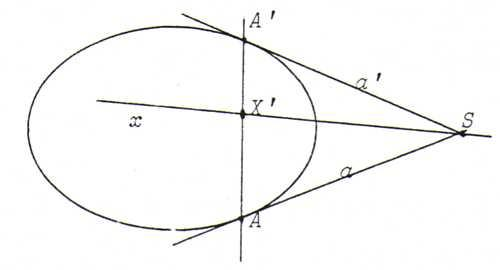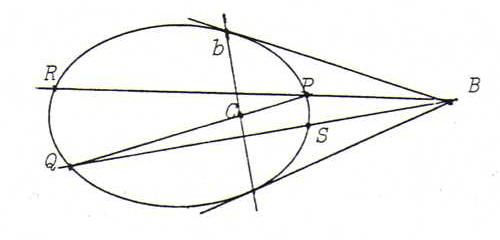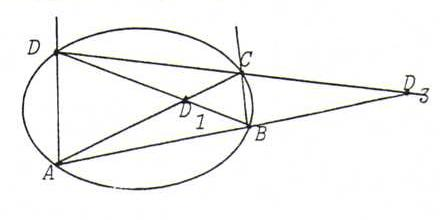### COURSE OF PROJECTIVE GEOMETRY

§ 25:

O92
Proof that the hint is valid:
Let S := a. x. Let a and a ' be the tangents to J through S. We assume aa '. Let A and A ' be the points of contact on a and a ', respectively.Since S lies on x, X lies on s (= AA ' ), the polar line of S.
Since X and X ' = s. x form a pair that's harmonically separating {A , A ' }, SX and x form a pair that's harmonically separating {a , a ' }.

Construction:
S := a. x. Construct a ' using O86. Construct the fourth harmonic y with x and {a , a ' }.
Let T := b. x, and let b ' be the other tangent to J through T. Let z be the the fourth harmonic with x and {b , b ' }.
Then X := y.z .

O93 Let b be the polar line of B, so that C lies on b.We have to prove: C = PQ.RS.
Proof: Let T := PQ.RS and U := RQ.PS. Then b = UT (see §22). So T = PQ.b = C.

O94 A diagonal of quadrilateral abcd is the line connecting opposite intersection points, for example a.d and b.c .Since a.d and b.c lie on D1D3, etcetera, quadrangle ABCD and quadrilateral abcd have the same diagonals.